# RF Tutorial Lesson 8: Designing an RF Bipolar Junction Transistor Amplifier with Lumped Matching Networks

 Tutorial Project: Designing an RF Bipolar Junction Transistor Amplifier with Lumped Matching Networks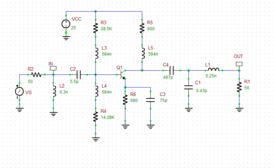Objective: In this project, the basic concepts of RF.Spice A/D are demonstrated, and a simple voltage divider is modeled and examined. Concepts/Features: Bipolar Junction Transistor Standard BJT Model RF BJT Model RF Amplifier DC Bias Analysis Stability Analysis Matching Network Voltage Gain Power Gain Minimum Version Required: All versions

## What You Will Learn

In this tutorial you will build an RF amplifier using a high frequency bipolar junction transistor (BJT) with lumped elements. First, you will examine the S-parameter model of the transistor and analyze its DC bias circuit. Then, you will calculate the port characteristics of the amplifier and verify its matching networks. Finally, you will run an AC frequency sweep analysis of the amplifier to characterize its voltage and gain performance.

## Comparing High Frequency & Low Frequency BJT Models

High frequency transistors are typically characterized by their S-parameters. The manufacturer data sheets of RF transistors usually contains tables of measured S-parameter data for various DC bias operating points over a certain range of frequencies. RF.Spice A/D has a large number of RF bipolar junction transistor (BJT) models with measured S-parameter tables for different combinations of collector-emitter voltages (VCE) and collector currents (IC). Most of RF.Spice's RF BJT devices also have counterpart standard BJT model that can be used for DC operating point analysis. The figures below shows the property dialog of the standard BJT model of BFG193 next to the property dialog of the RF BJT model of BFG193 measured at VCE = 10V and IC = 10mA. You will use this transistor for the amplifier design of this tutorial lesson.The property dialog of the standard BFG193 BJT model.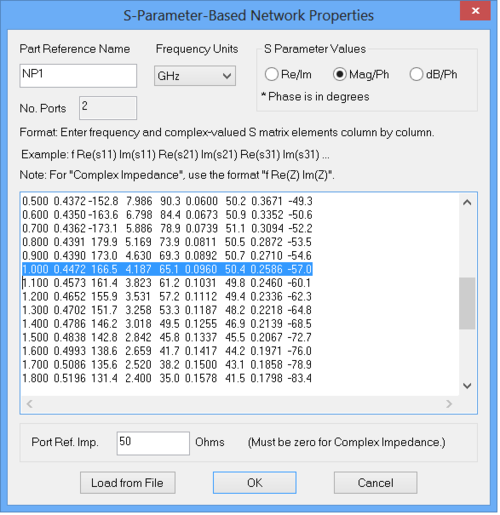The property dialog of the RF BFG193 BJT model measured at VCE = 10V and IC = 10mA.

## Examining the High Frequency BJT Model

You will start this tutorial lesson by testing the S-parameters of BFG193. Open the Parts Bin of the Toolbox and search for BFG193. You can search alphabetically or by function under Active > Transistor > NPN > RF. Place an instance of this BJT on the schematic and place two Net Markers (keyboard shortcut: Alt+N) to designate the base and collector of the BJT as the input and output of your circuit as shown in the opposite figure. Ground the emitter of the BJT.The symbols of the standards BJT and its RF counterpart with an S-parameter model are slightly different.

Run a Network Analysis Test of your simple two-port RF circuit.

 Start Frequency 500Meg 1500Meg 10Meg Linear S Cartesian (Amp-Phase) with Decibels

Set the start and stop frequencies of the sweep to 500MHz and 1500MHz, respectively, with a linear frequency step size of 10MHz. Choose "S" parameters on a Cartesian graph with both amplitude and phase and a dB scale. The figure below shows the S-parameter results with magnitudes in dB and phases in degrees. Use the tracking crosshairs of the graph window to read the value of |s21| at 1GHz. The reading at the bottom of the screen shows a value of 12.442dB, which corresponds to 4.187 as you can see from the highlighted row in the S-parameter table of the BJT's property dialog.Graph of magnitude and phase of s11, s21, s12 and s22-parameters of BFG193 over the frequency range 500MHz - 1500MHz.

## Building the BJT Amplifier and Testing Its DC Bias Circuit

The following is a list of parts needed for this part of the tutorial lesson:

Part Name Part Type Part Value
VCC DC Voltage source 25V
VS AC Voltage source 1V
Q1 NPN BJT Defaults: Standard BFG193 Model
XTL2 Generic T-Line Z0 = 50, eeff = 1, len = 18
XTLO1 Generic Open Stub Z0 = 50, eeff = 1, len = 59.55
R1 - R2 Resistor 50
R3 Resistor 28.5k
R4 Resistor 14.28k
R5 Resistor 800
R6 Resistor 680
L1 Inductor 5.25n
L2 Inductor 6.3n
L3 - L5 Inductor 584n
C1 Capacitor 0.43p
C2 Capacitor 5.5p
C3 Capacitor 75p
C4 Capacitor 493p
IN Net Marker N/A
OUT Net Marker N/A

Place and connect all the parts as shown in the above figure. Note that for this part you will use the standard BJT model with the "Ideal Forward Beta (BF)" parameter. You need the standard model to perform a DC operating point analysis. R3, R4, R5 and R6 are DC bias resistors. The resistors R3 and R4 acts as a voltage divider for the power supply VCC. R5 and R6 control and determine the collector and emitter currents, respectively. L1 and L2 are tuning inductors and C1 and C2 are tuning capacitors. The RF chokes L3, L4 and L5 pass the DC currents but block the AC signal and effectively remove the resistors R3, R4 and R5 from the RF analysis. The bypass capacitor C3 shorts the resistor R4 in the AC analysis. The DC blocking capacitor C4 isolates the DC bias circuitry form the load but acts as a short circuit for AC analysis. Note that for this circuit, the tuning capacitor C2 also acts as a DC blocking capacitor which isolates the bias circuitry from the AC source.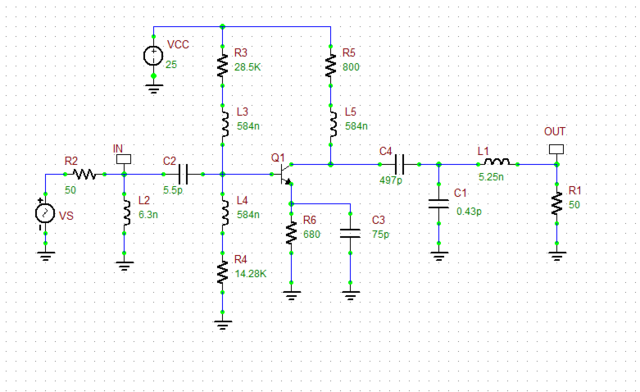The RF BJT Amplifier with standard BJT model for DC bias analysis.

The DC bias circuit has been designed to generate a collector current of IC = 10mA with a collector-emitter voltage of VCE = 10V. For this reason, you will use the S-parameter RF model BFG193v10v10mA in the next part. Run a DC Bias Test of your BJT amplifier to find its DC operating point parameters.The results of DC Bias Test of the BJT Amplifier using BFG193's standard BJT Model.

From the results table, you will see:

IC = 10.087mA

VCE = VQC - VQE = 16.933 -6.911 = 10.022V

which validate the DC bias design goals. You can also see all the DC voltages and currents by running a live simulation of the circuit and enabling circuit animation on the Schematic Editor.

## Stability Analysis of the BJT Transistor & RF Design Strategy

Before designing the matching networks, first you have to perform a stability analysis of the RF BFG193 transistor at the operating frequency of 1GHz. The table below gives the S-parameter values of this BJT at 1GHz:

S-parameter Mag-Phase Real-Imag
s11 0.4472 ∠166.5° -0.4348 +j 0.1044
s21 4.187 ∠65.1° 1.7629 + j3.7978
s12 0.0960 ∠50.4° 0.0612 + j0.0740
s22 0.2586 ∠-57.0° 0.1408 - j0.2169

To perform a K-Δ stability test of the BJT, we need to calculate the following quantities:

$\left| \Delta \right| = \left| s_{11}s_{22} - s_{12}s_{21} \right| = \left| 0.1346 - j0.2539 \right| = 0.2873$

$K = \frac {1 - |s_{11}|^2 - |s_{22}|^2 + |\Delta |^2 } { 2|s_{12}s_{21}| } = 1.0144$

It can be seen that Rollet's condition, K > 1 and |Δ| <1, is satisfied at 1GHz. So the BFG193 BJT is unconditionally stable at 1GHz with a DC operating condition of VCE = 10V and IC = 10mA.

Although the BJT is not unilateral (s12 ≠ 0), you will adopt a unilateral design strategy and will verify your design outcome later. For this purpose, you will set

$\Gamma_S = s_{11}^\ast = -0.4348 -j 0.1044 = 0.4472 \angle -166.5^o$

$\Gamma_L = s_{22}^\ast = 0.1408 + j0.2169 = 0.2586 \angle 57.0^o$

where ΓS and ΓL are the source and load reflection coefficients looking to the left and right of the transistor.

## Designing Input and Output Matching Networks

Based on the source and load reflection coefficients established in the previous section, you can now design LC matching network sections to be inserted between the base of the BJT and the voltages source and between the collector of the BJT and the load. For the input matching network you will use a shunt inductor L2 and a series capacitor C2 in conjunction with the source resistor R2. Note that the AC voltage source must be shorted for the calculation of the input reflection coefficient. Set up a simple circuit as shown in the figure below and assign a single port between the free node of the series capacitor and the ground. Run a Network Analysis Test of this circuit with the following parameters. From the results, note that at 1GHz the required source reflection coefficient has been achieved.

 Start Frequency 500Meg 1500Meg 500Meg Linear S Cartesian (Amp-Phase) with Decibels unchecked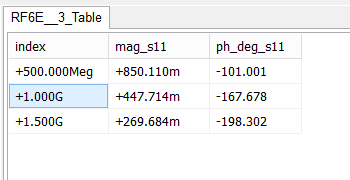The computed results of source reflection coefficient for the input matching network at 1GHz.

Next, you will use a shunt capacitor C1 and a series inductor L1 in conjunction with the load resistor R1 for the output matching network. Set up another simple circuit as shown in the figure below and assign a single port between the top node of the shunt capacitor and the ground. Run a Network Analysis Test of this circuit using the same parameters table as above. As you can see from the S11 parameter table, the required load reflection coefficient has been achieved.The computed results of load reflection coefficient for the output matching network at 1GHz.

## Running a Network Analysis of the BJT Amplifier

At this point, your BJT amplifier circuit is ready for an overall Network Analysis Test. Replace the standard BFG193 model called Q1 with RF BJT model BFG193v10v10mA called NP1. Place the "IN" marker right after the source resistor, and place the "OUT" marker at the load resistor. Then remove the voltage source as well as the source and load resistors R2 and R1. The figure below shows this circuit with the source and load sections removed:For a two-port Network Analysis Test, you should remove the voltage source, source resistance and load resistance from your circuit.The RF BJT Amplifier with S-parameter RF BJT model set up for network analysis.

Run a Network Analysis Test of the true amplifier circuit independent of the source and load with the parameters specified below:

 Start Frequency 500Meg 1500Meg 10Meg Linear S Cartesian (Amplitude) with Decibels

The figure below shows the results for S11, S21, S12 and S22 parameters. A tracking crosshair has been used to read the value of |s21| at 1GHz. This value is 15.4dB. Note that the total input reflection coefficient and the total output reflection coefficient are both equal to or below -10dB at 1GHz and the overall |s12| of the circuit is negligible. This validates your design strategy based on the unilateral assumption. You will see in the next section that the computed values of |s21| (insertion loss or gain) is very close to the computed value of the power gain from the source to the load.The graph of magnitude of S11, S21, S12 and S22 parameters of the BJT amplifier circuit without the source and load sections.

## Running an AC Frequency Sweep Test of the BJT Amplifier

In the last part of this tutorial lesson, you will run an AC Sweep Test of your BJT amplifier circuit to examine the input and output voltages and find the voltage gain and power gain of your amplifier. First, you need to put back the AC voltage source VS, the source resistor R2 and the load resistor R1 in your amplifier circuit. To compute the source and load currents IS and IL, you can place two ammeters (keyboard shortcut: Alt+Y), one between the AC voltage source and the source resistor, and the other just before the load resistor as shown in the figure below.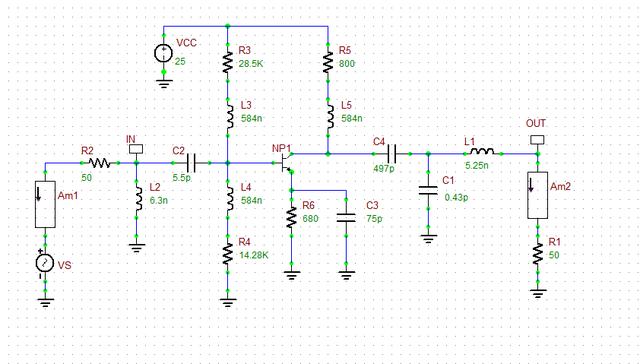The RF BJT Amplifier with S-parameter RF BJT model for AC frequency sweep test including source and load ammeters.

Run an AC frequency sweep with the parameters specified below:

 Start Frequency 500Meg 1500Meg 10Meg Linear vm(IN), vm(OUT)

You will get input and output voltage graphs like the ones shown below. Enable the tracking crosshairs and read the values at 1GHz. Note that when you have multiple plots, the tracking crosshairs read the value of the highlighted (selected) plot. The input and output voltage readings are 535mV and 2.941V, respectively. Therefore, the voltage gain must be 20*log10(v(OUT)/v(IN)) = 20*log10(5.5) = 14.8dB. The input voltage value shows that the default 1V voltage of the source VS has been almost equally divided between the source resistor and the amplifier circuit.The graph of the magnitude of input and output voltages of the BJT amplifier on a linear scale.

To measure the power gain, we define input and output powers as the source and load powers:

$P_{in} = P_S = \frac{1}{2} R_S |I_S|^2$

$P_{out} = P_L = \frac{1}{2} R_L |I_L|^2$

$G_P = \frac{P_L}{P_S} = \left| \frac{I_L}{I_S} \right| ^2$

Note that RS = RL = 50Ω. Before closing this tutorial lesson, you will calculate the voltage and power gains of your BJT amplifier. For this purpose, you will define "Custom Output Plots". In the AC Sweep Test Panel, click the "Preset Graphs..." button to open the Edit Plot List dialog. Define two new custom output plots with the names "Voltage_Gain" and "Power_Gain" as shown in the figures below. Use the following definitions:

Voltage_Gain: 20*log10(abs(v(out)/v(in)))

Power_Gain: 20*log10(abs(i(am2)/i(am1)))

Note that a dB-scale definition has been used. Remove the voltage amplitudes vm(in) and vm(out) from the graph plot list in the Edit Plot List dialog.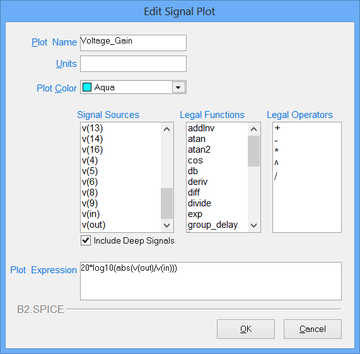Definition of Voltage Gain in Edit Signal Plot dialog.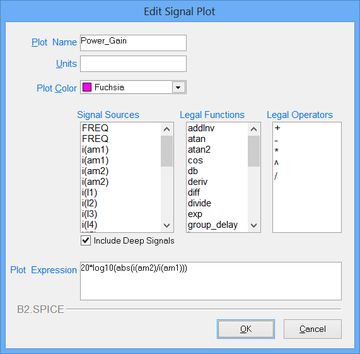Definition of Power Gain in Edit Signal Plot dialog.

Run the AC sweep test one more time to plot the voltage and power gain as a function of frequency:

 Start Frequency 500Meg 1500Meg 10Meg Linear Custom: Voltage_Gain, Power_Gain

Using the tracking crosshairs read the values of the two gains at 1GHz. The power gain at this frequency is 15.12dB and the voltage gain is 14.83dB, which agrees perfectly with your earlier calculation. Note that power gain rises to a peak around 825MHz, while the voltage gain rises monotonically. However, both input and output voltages of the amplifier start to decrease with increasing frequency. At 1.5GHz, the output voltage is about 1.5V.The graph of the voltage and power gains of the BJT amplifier vs. frequency.